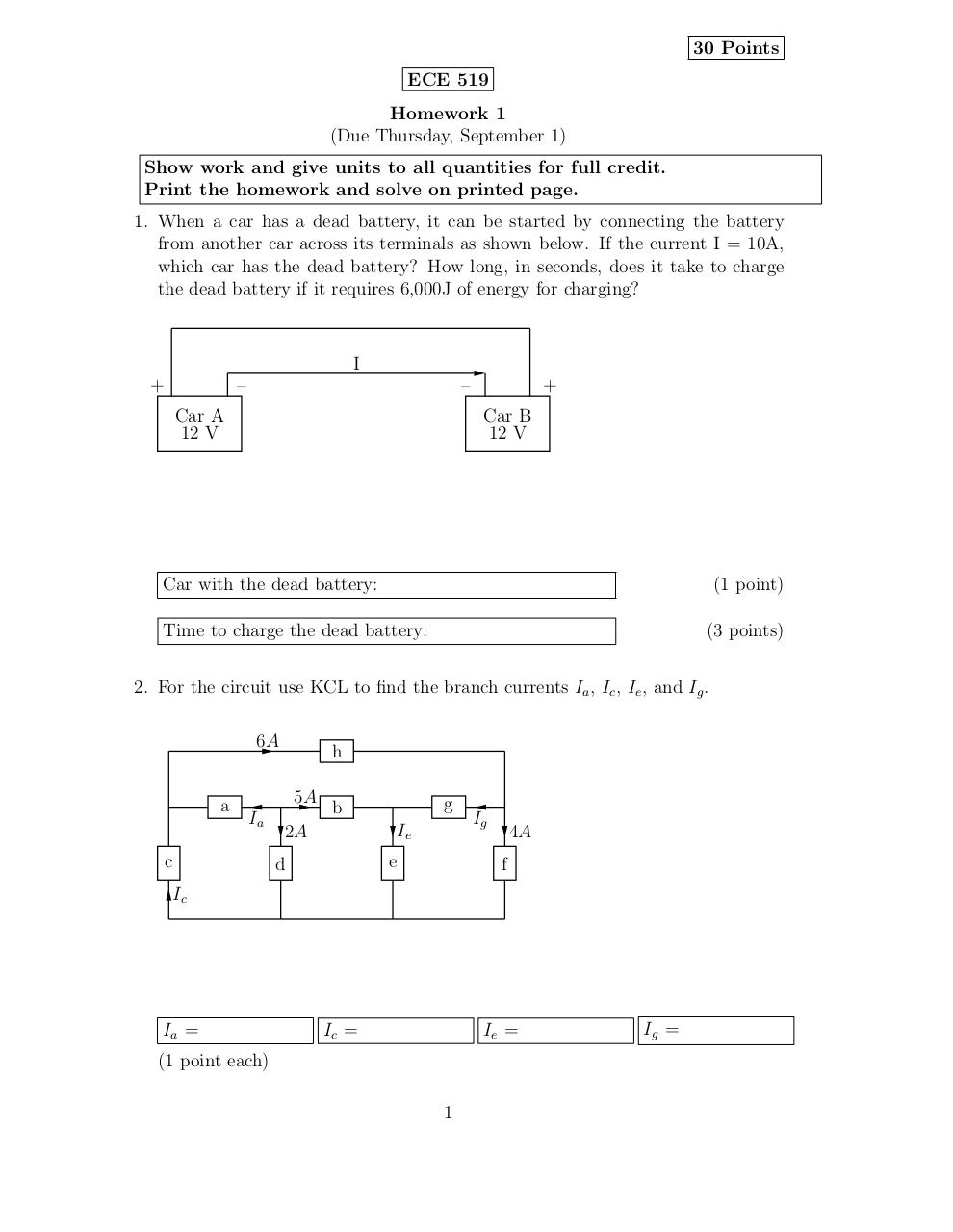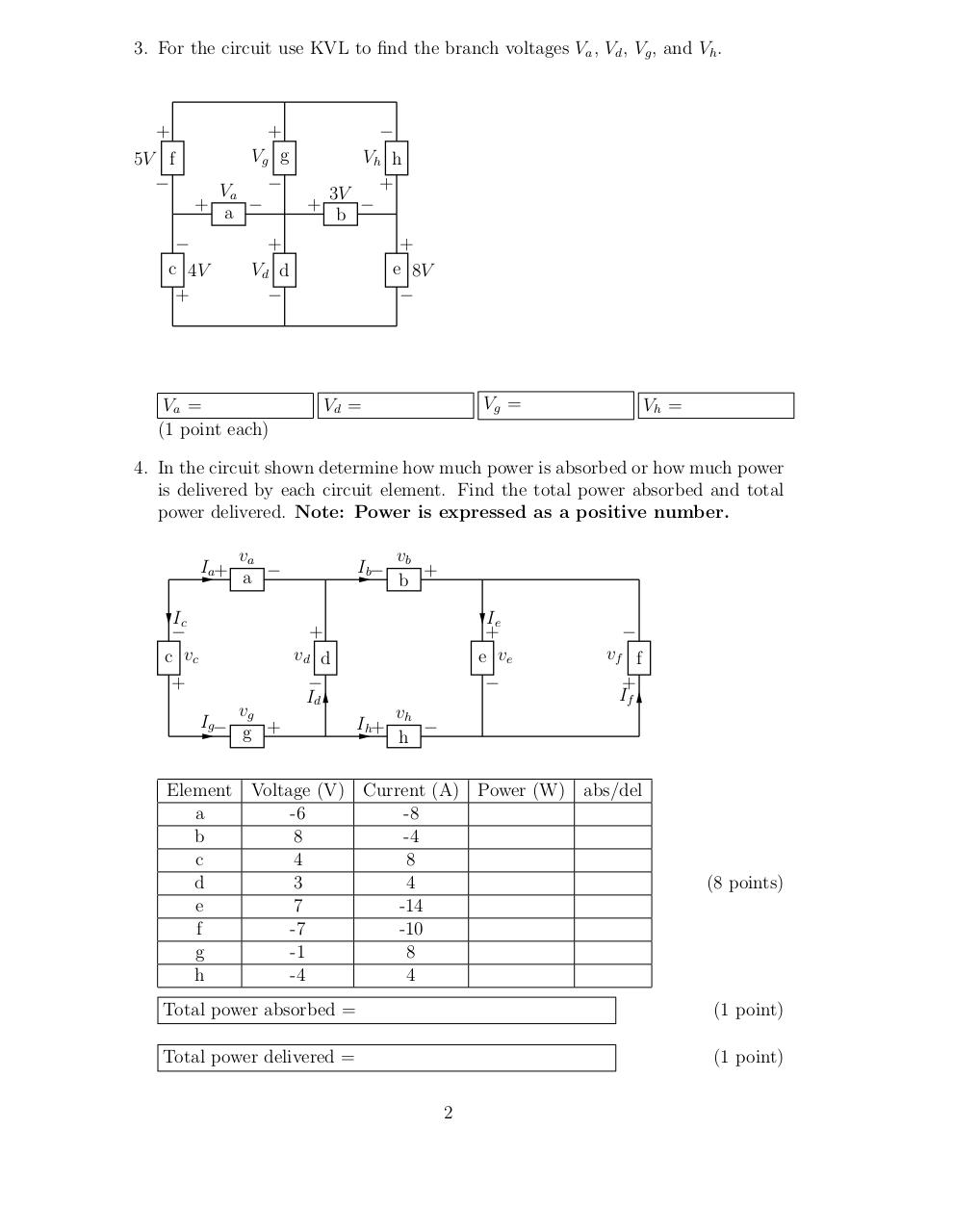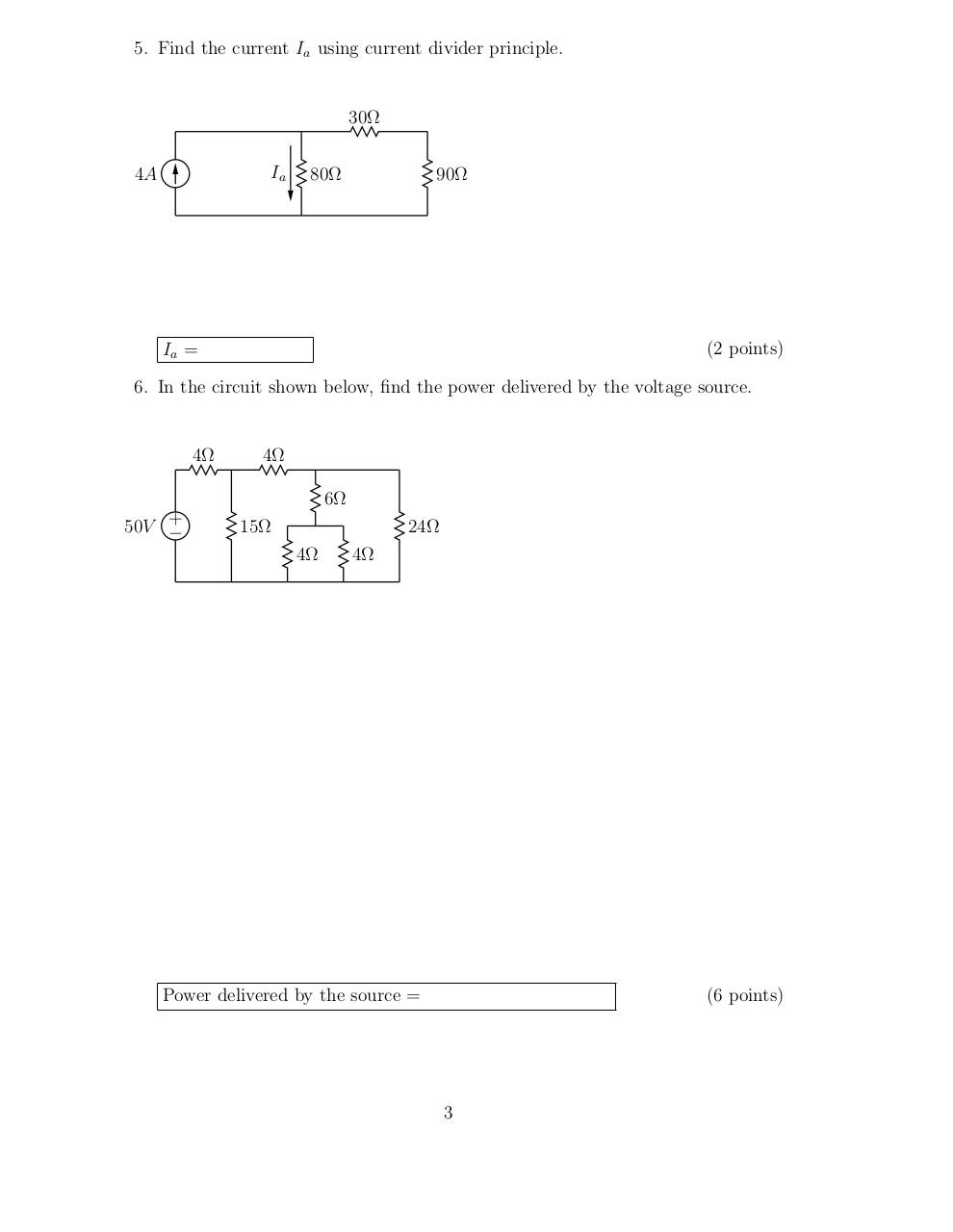# hw1 f16 .pdf

### File information

Original filename: hw1_f16.pdf
Title: hw1_f16.dvi

This PDF 1.4 document has been generated by dvips(k) 5.94b Copyright 2004 Radical Eye Software / AFPL Ghostscript 8.54, and has been sent on pdf-archive.com on 28/08/2016 at 05:31, from IP address 70.179.x.x. The current document download page has been viewed 341 times.
File size: 28 KB (3 pages).
Privacy: public file

hw1_f16.pdf (PDF, 28 KB)

### Document preview

30 Points
ECE 519
Homework 1
(Due Thursday, September 1)
Show work and give units to all quantities for full credit.
Print the homework and solve on printed page.
1. When a car has a dead battery, it can be started by connecting the battery
from another car across its terminals as shown below. If the current I = 10A,
which car has the dead battery? How long, in seconds, does it take to charge
the dead battery if it requires 6,000J of energy for charging?

I
+

+

Car A
12 V

Car B
12 V

(1 point)
(3 points)

Time to charge the dead battery:

2. For the circuit use KCL to find the branch currents Ia , Ic , Ie , and Ig .
6A
a

h
5A

Ia

c

g

b
Ie

2A

e

d

Ig

4A
f

Ic

Ia =

Ic =

Ie =

(1 point each)
1

Ig =

3. For the circuit use KVL to find the branch voltages Va , Vd , Vg , and Vh .

+
5V f

+
g
Vg
Vh h
+

Va
3V

+ a −
+
b

c 4V
+

+
Vd d

Va =
(1 point each)

+
e 8V

Vg =

Vd =

Vh =

4. In the circuit shown determine how much power is absorbed or how much power
is delivered by each circuit element. Find the total power absorbed and total
power delivered. Note: Power is expressed as a positive number.
Ia+

va
a −

Ic

c vc
+
Ig−
Element
a
b
c
d
e
f
g
h

vg
g +

Ib−

vb
+
b
Ie
+
e ve

+
vd d

Id
Ih+

vh

h

Voltage (V) Current (A) Power (W)
-6
-8
8
-4
4
8
3
4
7
-14
-7
-10
-1
8
-4
4

vf f
+
If

abs/del

(8 points)

Total power absorbed =

(1 point)

Total power delivered =

(1 point)
2

5. Find the current Ia using current divider principle.

30Ω
Ia

4A

80Ω

90Ω

(2 points)

Ia =

6. In the circuit shown below, find the power delivered by the voltage source.

4Ω

4Ω
6Ω

+
50V −

15Ω

24Ω
4Ω

4Ω

Power delivered by the source =

(6 points)

3#### HTML Code

Copy the following HTML code to share your document on a Website or Blog

#### QR Code### Related keywords